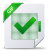# A State of a Modula Evolving Algebra

## Cours Algebraic Operational Semantics and Modula-2*, tutoriel & guide de travaux pratiques en pdf.

A State of a Modula Evolving Algebra

Since an evolving algebra for Modula reflects a given program, we give a sample program Prog which will allow us to supply concrete examples of the universes and functions comprising an algebra. The sample program appears in Figure 1.
We now describe the universes, functions and relations that comprise an evolving algebra M(Prog) for Prog. We also mention in passing those components which might be present in an algebra for a Modula program different from Prog but which are unnecessary to an account of Prog’s ideal machine. We use evolving algebra and dynamic structure interchangeably in our account. All universes of a dynamic structure have the equality relation defined on them.
Integers
Prog declares a record type, Vertex, that includes a field of type integer. Therefore, a dynamic structure M(Prog) will include a universe int consisting of all the integers in
MODULE Prog; FROM Storage IMPORT ALLOCATE; FROM InOut IMPORT ReadInt, Done, WriteInt, WriteLn; TYPE Link = POINTER TO Vertex; Vertex = RECORD datum: INTEGER; left, right: Link END; VAR r, tree: Link;
PROCEDURE Insert(item: Link; VAR subtree: Link); BEGIN IF subtree = NIL THEN subtree := item; subtree

.left := NIL;
subtree

.right := NIL ELSEIF item

.datum < subtree

.datum THEN
Insert(item, subtree

.left)
ELSE Insert(item, subtree

.right)
ENDIF END Insert;
PROCEDURE Print(subtree: Link); BEGIN IF subtree

.left

NIL THEN Print (subtree

.left);
WriteInt(subtree

.datum,6);Writeln;
IF subtree

.right

NIL THEN Print(subtree

.right)
END Print;
BEGIN tree := NIL; NEW(r); ReadInt(r

.datum);
WHILE Done DO Insert(r, tree); NEW(r); ReadInt(r

.datum)
END; IF tree

NIL THEN Print(tree) END Prog.
the interval [MinInt, MaxInt]. MinInt and MaxInt are distinguished elements of int. The universe int comes equipped with the usual ordering and the partial arithmetic operations +, −, × , quotient, and remainder.

Boolean

A structure M(Prog) will include a universe bool = {true, false} equipped with the usual Boolean operations and ordered such that false < true. The binary Boolean operations are used only in transition rules. In Modula, the binary Boolean operations appear in a « conditional » form in which both an operation’s arguments are not always evaluated. Consequently, their semantics are given by the transition rules that govern sequencing through the parse trees of Boolean expressions.

### Other Basic Types

The other universes a Modula structure may comprise are a finite ordered set of characters char, an initial segment of the natural numbers card, and a finite set of real numbers real. In general, char and its ordering relation differ among Modula structures, but char must contain the upper case letters of the Roman alphabet, the digits 0, . . . , 9, and certain punctuation marks (cf. The Modula Report ). The universe char is equipped with operations, Ord and Char, which give the position in the ordering of one of its elements and the element corresponding to a position in the ordering, respectively. The universe card includes those natural numbers less than or equal to MaxCard, where MaxCard is a constant that differs among Modula structures. The usual ordering and (partial) arithmetic operations are defined on card. We omit a description of real.

#### The Parse Tree

The parse tree of Prog is represented by a universe called parsetree with a relatively rich structure plus some additional functions from and to parsetree. The elements of parsetree are the nodes of the parse tree. The partial functions Child1, Child2, . . . map an element of parsetree to its first, second, etc. child, if it has one. The function Children indicates how many children a node possesses. The function Parent maps an element of parsetree to its parent node, if it has one.Télécharger le cours complet